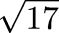Next Continued Fraction Arithmetic 46

# Truncation

• This is probably the most important property of continued fractions

• Suppose you truncate the continued fraction for n

• You get a very good rational approximation for n

• (In the sense that no fraction with a smaller denominator is closer)

• Consider= [4; 8, 8, 8, 8, ...] = 4.12310562561...:

```                     = 4/1           = 4
[4; 8]             = 33/8          = 4.125
[4; 8, 8]          = 268/65        = 4.1230769...
[4; 8, 8, 8]       = 2177/528      = 4.12310606
[4; 8, 8, 8, 8]    = 17684/4289    = 4.123105619...
```
• 268/65 has a denominator smaller than 4.12 = 412/100

• But it is more than 100 times as accurate

• Even 33/8 is more accurate than 412/100

• Conclusion: You needn't calculate the whole expansion of a continued fraction

• If you stop early, you get a good approximation

 Next Back